Polar representation

Chapter 5 Class 11 Complex Numbers
Concept wise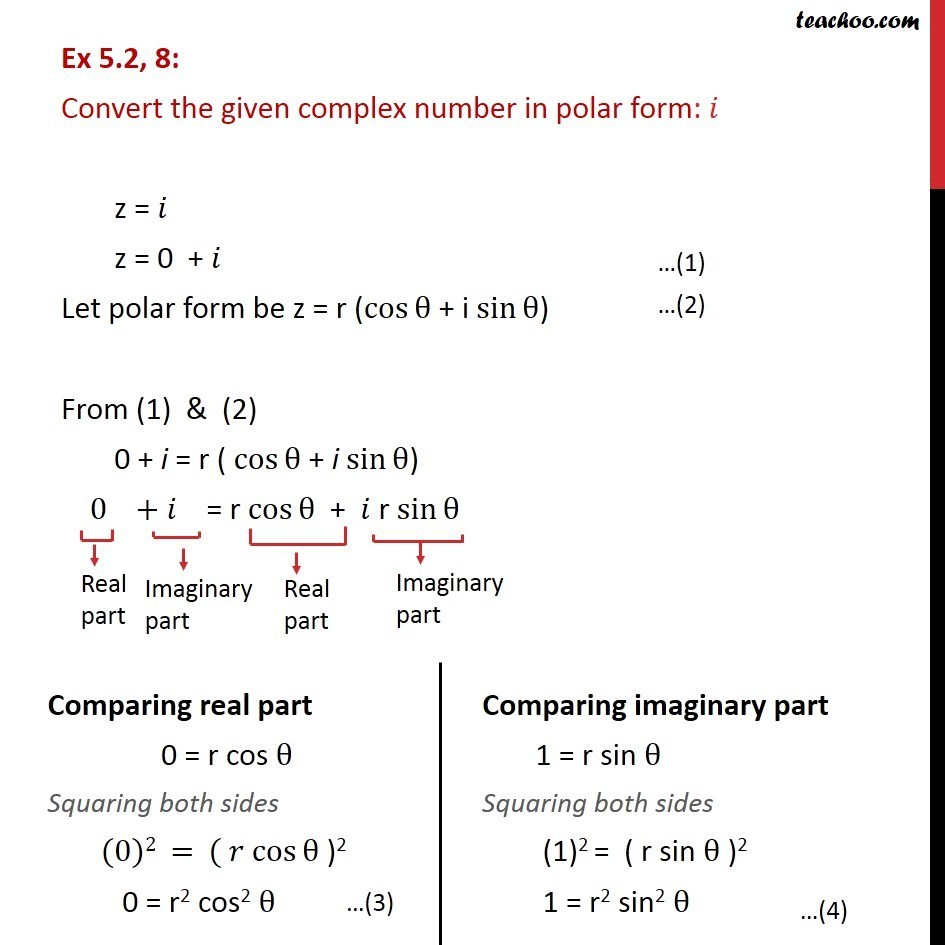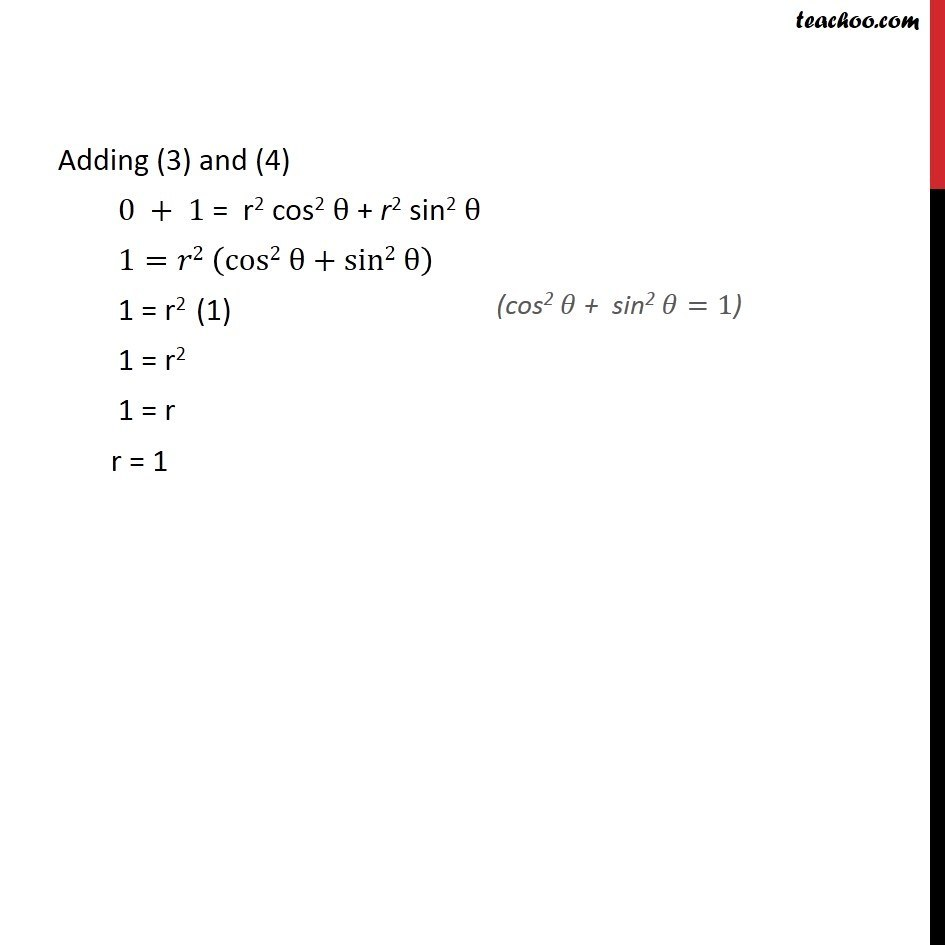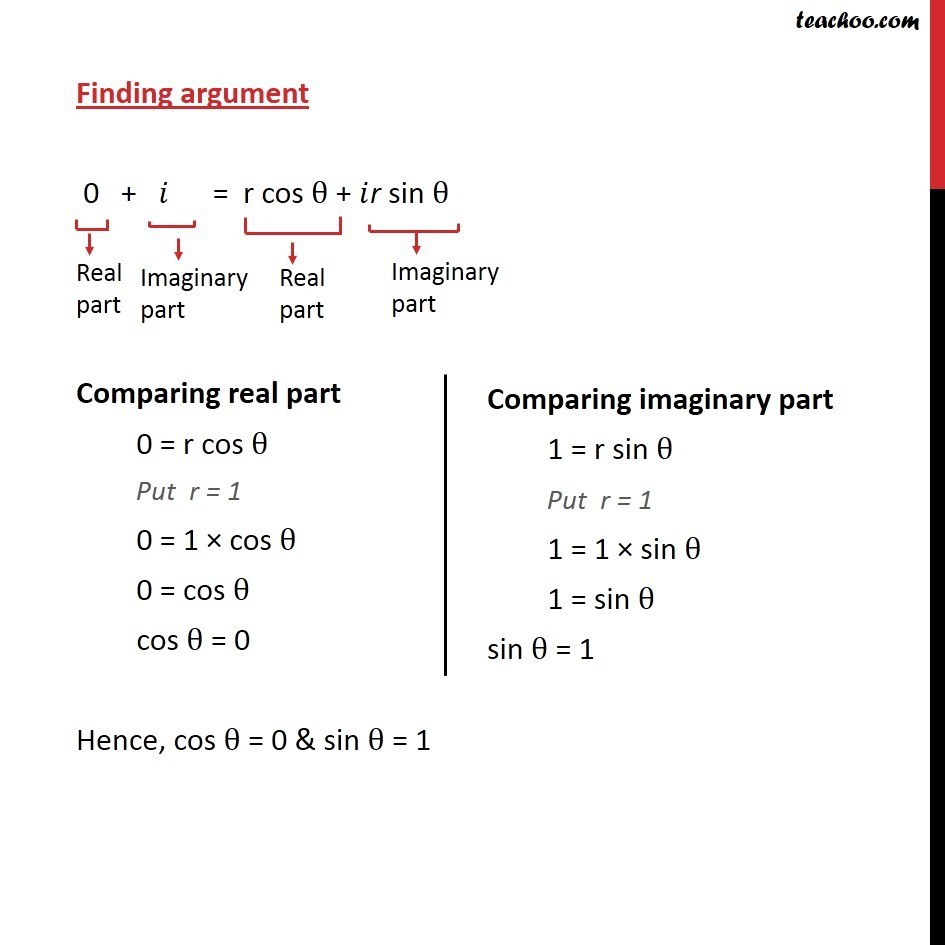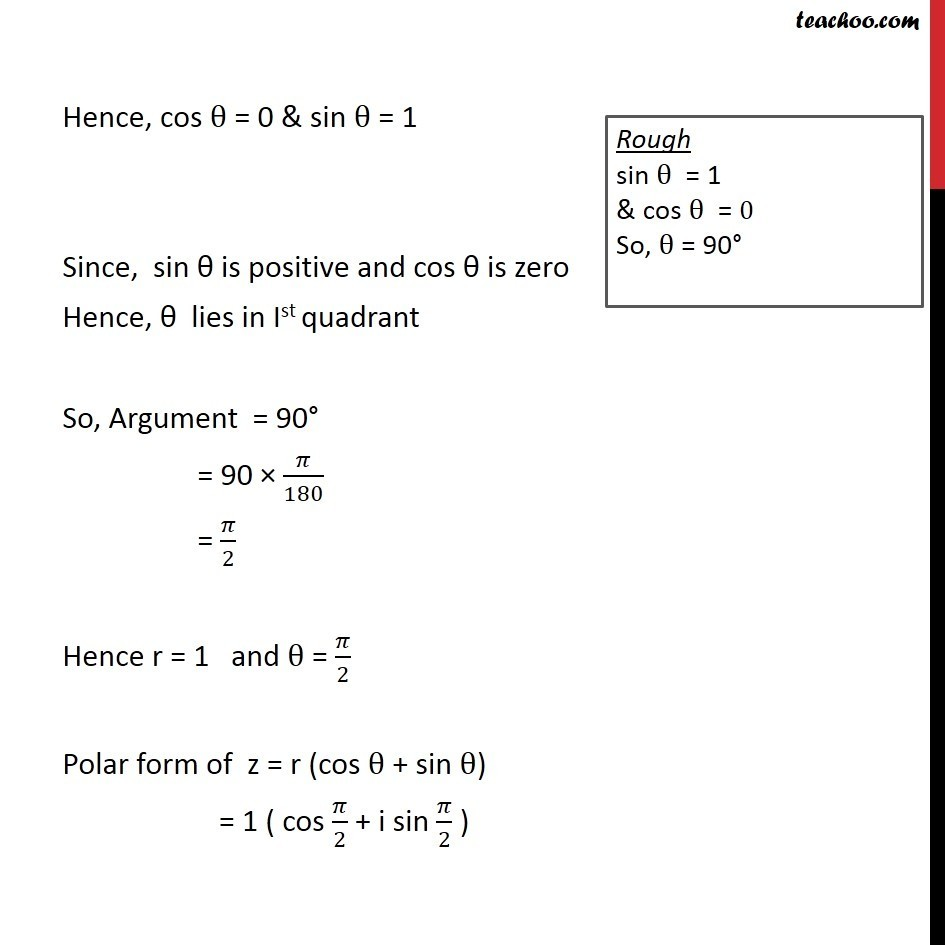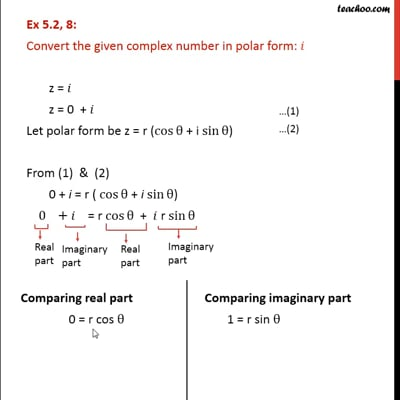This video is only available for Teachoo black users

Introducing your new favourite teacher - Teachoo Black, at only ₹83 per month

### Transcript

Ex 5.2, 8: Convert the given complex number in polar form: 𝑖 z = 𝑖 z = 0 + 𝑖 Let polar form be z = r (cos⁡θ + i sin⁡θ) From (1) & (2) 0 + i = r ( cos⁡θ + i sin⁡θ) 0 + 𝑖 = r〖 cos〗⁡θ + 𝑖 r sin⁡θ Adding (3) and (4) 0 + 1 = r2 cos2 θ + r2 sin2 θ 1=𝑟2 (cos2 θ+sin2 θ) 1 = r2 (1) 1 = r2 1 = r r = 1 Finding argument 0 + 𝑖 = r cos θ + 𝑖r sin θ Comparing real part 0 = r cos θ Put r = 1 0 = 1 × cos θ 0 = cos θ cos θ = 0 Hence, cos θ = 0 & sin θ = 1 Hence, cos θ = 0 & sin θ = 1 Since, sin θ is positive and cos θ is zero Hence, θ lies in Ist quadrant So, Argument = 90° = 90 × 𝜋/180 = 𝜋/2 Hence r = 1 and θ = 𝜋/2 Polar form of z = r (cos θ + sin θ) = 1 ( cos 𝜋/2 + i sin 𝜋/2 )